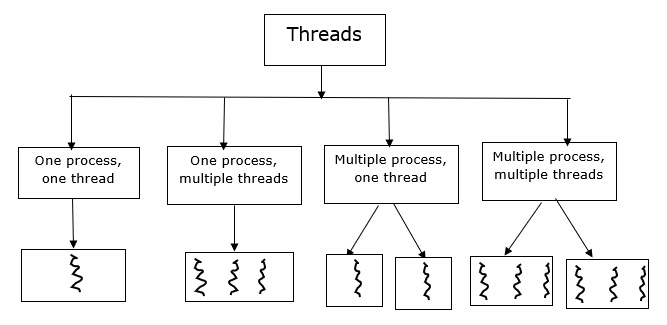# What is a multithreading model in OS?

Multithreading is a process divided into a number of smaller tasks. Each task is represented or called it as a “Thread”. That means a thread is a lightweight of process. A number of such threads within a process executes at a time is called “multithreading”.

Based on the functionality, threads are divided into four categories and these are given below −

• Multiple processes, one thread per process.

• Multiple processes, multiple threads per process.

Let us see how the above functionality represents in the pictorial form −• One process, one thread − It is a traditional approach, in which the process maintains only one thread, so it is called a single threaded approach. For example − MS-DOS OS supports one thread, one process approach.

• One process, multiple threads − Here, a process is divided into a number of threads. For example − JAVA runtime environment.

• Multiple processes, one thread per process − An Operating system supports multiple user processes but only supports one thread per process. For example − UNIX operating system.

• Multiple processes, multiple threads per process − Here, each process is divided into a number of threads. For example − Windows 2000, Solaris LINUX.

### Differences

The major differences between process and threads are as follows −